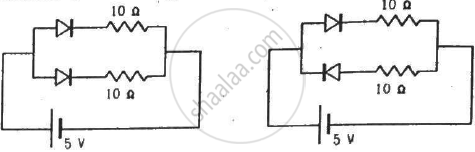Advertisement Remove all ads

# Find the Current Through the Battery in Each of the Circuits Shown in Figure. - Physics

Short Note

Find the current through the battery in each of the circuits shown in figure.(Assume that the resistance of each diode is zero in forward bias and is infinity in reverse bias.)

Advertisement Remove all ads

#### Solution

We know that when a diode is forward biassed, it has zero resistance ideally. So, it can be replaced by a short circuit. When a diode is reverse biassed, it has infinite resistance ideally. So, it can be replaced by an open circuit.

(a) In the given circuit diagram, both diodes are forward biassed. So, the resistance of both of them is zero. Thus, the diode resistance is zero.

$i = \frac{5}{\frac{10 \times 10}{10 + 10}} = \frac{5}{5} = 1 A$

(b) One diode is forward biassed and the other is reverse biassed. The reverse-biassed diode is replaced by an open circuit, so no current flows through this branch.
The current passes through the forward-biassed diode only.

$i = \frac{V}{R_{net}} = \frac{5}{10} = 0 . 5 A$

Concept: p-n Junction
Is there an error in this question or solution?
Advertisement Remove all ads

#### APPEARS IN

HC Verma Class 11, Class 12 Concepts of Physics Vol. 2
Chapter 23 Semiconductors and Semiconductor Devices
Q 27 | Page 420
Advertisement Remove all ads
Advertisement Remove all ads
Share
Notifications

View all notifications

Forgot password?